# NCERT solutions for Mathematics Exemplar Class 9 chapter 10 - Circles [Latest edition]

#### Chapters## Chapter 10: Circles

Exercise 10.1Exercise 10.2Exercise 10.3Exercise 10.4
Exercise 10.1 [Pages 99 - 101]

### NCERT solutions for Mathematics Exemplar Class 9 Chapter 10 Circles Exercise 10.1 [Pages 99 - 101]

Exercise 10.1 | Q 1 | Page 99

AD is a diameter of a circle and AB is a chord. If AD = 34 cm, AB = 30 cm, the distance of AB from the centre of the circle is ______.

• 17 cm

• 15 cm

• 4 cm

• 8 cm

Exercise 10.1 | Q 2 | Page 99

In figure, if OA = 5 cm, AB = 8 cm and OD is perpendicular to AB, then CD is equal to ______.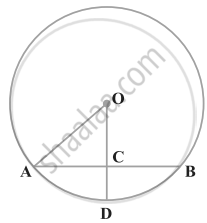• 2 cm

• 3 cm

• 4 cm

• 5 cm

Exercise 10.1 | Q 3 | Page 99

If AB = 12 cm, BC = 16 cm and AB is perpendicular to BC, then the radius of the circle passing through the points A, B and C is ______.

• 6 cm

• 8 cm

• 10 cm

• 12 cm

Exercise 10.1 | Q 4 | Page 99

In figure, if ∠ABC = 20º, then ∠AOC is equal to ______.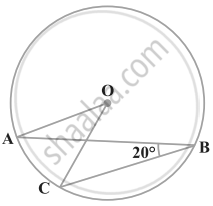• 20º

• 40º

• 60º

• 10º

Exercise 10.1 | Q 5 | Page 99

In figure, if AOB is a diameter of the circle and AC = BC, then ∠CAB is equal to ______.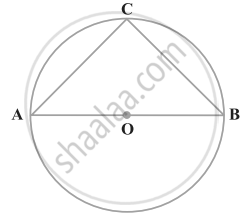• 30º

• 60º

• 90º

• 45º

Exercise 10.1 | Q 6 | Page 100

In figure, if ∠OAB = 40º, then ∠ACB is equal to ______.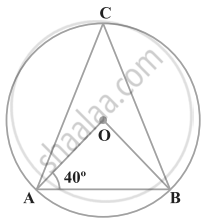• 50º

• 40º

• 60º

• 70°

Exercise 10.1 | Q 7 | Page 100

In figure, if ∠DAB = 60º, ∠ABD = 50º, then ∠ACB is equal to ______.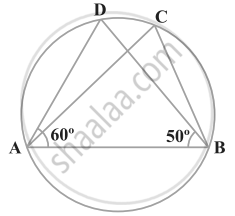• 60º

• 50º

• 70º

• 80º

Exercise 10.1 | Q 8 | Page 100

ABCD is a cyclic quadrilateral such that AB is a diameter of the circle circumscribing it and ∠ADC = 140º, then ∠BAC is equal to ______.

• 80º

• 50º

• 40º

• 30º

Exercise 10.1 | Q 9 | Page 100

In figure, BC is a diameter of the circle and ∠BAO = 60º. Then ∠ADC is equal to ______.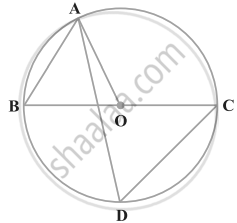• 30º

• 45º

• 60º

• 120º

Exercise 10.1 | Q 10 | Page 101

In figure, ∠AOB = 90º and ∠ABC = 30º, then ∠CAO is equal to ______.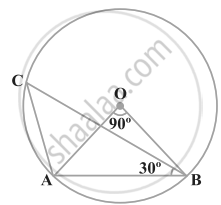• 30º

• 45º

• 90º

• 60º

Exercise 10.2 [Pages 101 - 102]

### NCERT solutions for Mathematics Exemplar Class 9 Chapter 10 Circles Exercise 10.2 [Pages 101 - 102]

#### State whether the following statement is True or False:

Exercise 10.2 | Q 1 | Page 101

Two chords AB and CD of a circle are each at distances 4 cm from the centre. Then AB = CD.

• True

• False

Exercise 10.2 | Q 2 | Page 101

Two chords AB and AC of a circle with centre O are on the opposite sides of OA. Then ∠OAB = ∠OAC .

• True

• False

Exercise 10.2 | Q 3 | Page 101

Two congruent circles with centres O and O′ intersect at two points A and B. Then ∠AOB = ∠AO′B.

• True

• False

Exercise 10.2 | Q 4 | Page 101

Through three collinear points a circle can be drawn.

• True

• False

Exercise 10.2 | Q 5 | Page 101

A circle of radius 3 cm can be drawn through two points A, B such that AB = 6 cm.

• True

• False

Exercise 10.2 | Q 6 | Page 102

If AOB is a diameter of a circle and C is a point on the circle, then AC2 + BC2 = AB2.

• True

• False

Exercise 10.2 | Q 7 | Page 102

ABCD is a cyclic quadrilateral such that ∠A = 90°, ∠B = 70°, ∠C = 95° and ∠D = 105°.

• True

• False

Exercise 10.2 | Q 8 | Page 102

If A, B, C, D are four points such that ∠BAC = 30° and ∠BDC = 60°, then D is the centre of the circle through A, B and C.

• True

• False

Exercise 10.2 | Q 9 | Page 102

If A, B, C and D are four points such that ∠BAC = 45° and ∠BDC = 45°, then A, B, C, D are concyclic.

• True

• False

Exercise 10.2 | Q 10 | Page 102

In figure, if AOB is a diameter and ∠ADC = 120°, then ∠CAB = 30°.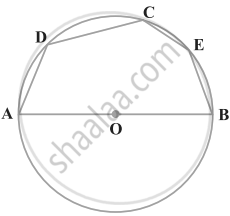• True

• False

Exercise 10.3 [Pages 103 - 105]

### NCERT solutions for Mathematics Exemplar Class 9 Chapter 10 Circles Exercise 10.3 [Pages 103 - 105]

Exercise 10.3 | Q 1 | Page 103

If arcs AXB and CYD of a circle are congruent, find the ratio of AB and CD.

Exercise 10.3 | Q 2 | Page 103

If the perpendicular bisector of a chord AB of a circle PXAQBY intersects the circle at P and Q, prove that arc PXA ≅ Arc PYB.

Exercise 10.3 | Q 3 | Page 103

A, B and C are three points on a circle. Prove that the perpendicular bisectors of AB, BC and CA are concurrent.

Exercise 10.3 | Q 4 | Page 103

AB and AC are two equal chords of a circle. Prove that the bisector of the angle BAC passes through the centre of the circle.

Exercise 10.3 | Q 5 | Page 103

If a line segment joining mid-points of two chords of a circle passes through the centre of the circle, prove that the two chords are parallel.

Exercise 10.3 | Q 6 | Page 103

ABCD is such a quadrilateral that A is the centre of the circle passing through B, C and D. Prove that ∠CBD + ∠CDB = 1/2∠BAD

Exercise 10.3 | Q 7 | Page 103

O is the circumcentre of the triangle ABC and D is the mid-point of the base BC. Prove that ∠BOD = ∠A.

Exercise 10.3 | Q 8 | Page 103

On a common hypotenuse AB, two right triangles ACB and ADB are situated on opposite sides. Prove that ∠BAC = ∠BDC.

Exercise 10.3 | Q 9 | Page 103

Two chords AB and AC of a circle subtends angles equal to 90º and 150º, respectively at the centre. Find ∠BAC, if AB and AC lie on the opposite sides of the centre.

Exercise 10.3 | Q 10 | Page 103

If BM and CN are the perpendiculars drawn on the sides AC and AB of the triangle ABC, prove that the points B, C, M and N are concyclic.

Exercise 10.3 | Q 11 | Page 103

If a line is drawn parallel to the base of an isosceles triangle to intersect its equal sides, prove that the quadrilateral so formed is cyclic.

Exercise 10.3 | Q 12 | Page 104

If a pair of opposite sides of a cyclic quadrilateral are equal, prove that its diagonals are also equal.

Exercise 10.3 | Q 13 | Page 104

The circumcentre of the triangle ABC is O. Prove that ∠OBC + ∠BAC = 90º.

Exercise 10.3 | Q 14 | Page 104

A chord of a circle is equal to its radius. Find the angle subtended by this chord at a point in major segment.

Exercise 10.3 | Q 15 | Page 104

In figure, ∠ADC = 130° and chord BC = chord BE. Find ∠CBE.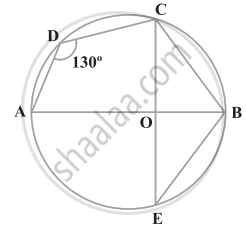Exercise 10.3 | Q 16 | Page 104

In figure, ∠ACB = 40º. Find ∠OAB.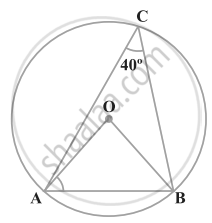Exercise 10.3 | Q 17 | Page 104

A quadrilateral ABCD is inscribed in a circle such that AB is a diameter and ∠ADC = 130º. Find ∠BAC.

Exercise 10.3 | Q 18 | Page 104

Two circles with centres O and O′ intersect at two points A and B. A line PQ is drawn parallel to OO′ through A(or B) intersecting the circles at P and Q. Prove that PQ = 2 OO′.

Exercise 10.3 | Q 19 | Page 104

In figure, AOB is a diameter of the circle and C, D, E are any three points on the semi-circle. Find the value of ∠ACD + ∠BED.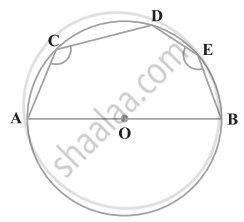Exercise 10.3 | Q 20 | Page 105

In figure, ∠OAB = 30º and ∠OCB = 57º. Find ∠BOC and ∠AOC.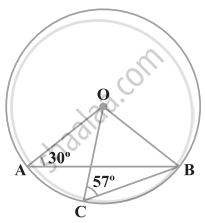Exercise 10.4 [Pages 106 - 107]

### NCERT solutions for Mathematics Exemplar Class 9 Chapter 10 Circles Exercise 10.4 [Pages 106 - 107]

Exercise 10.4 | Q 1 | Page 106

If two equal chords of a circle intersect, prove that the parts of one chord are separately equal to the parts of the other chord.

Exercise 10.4 | Q 2 | Page 106

If non-parallel sides of a trapezium are equal, prove that it is cyclic.

Exercise 10.4 | Q 3 | Page 106

If P, Q and R are the mid-points of the sides BC, CA and AB of a triangle and AD is the perpendicular from A on BC, prove that P, Q, R and D are concyclic.

Exercise 10.4 | Q 4 | Page 106

ABCD is a parallelogram. A circle through A, B is so drawn that it intersects AD at P and BC at Q. Prove that P, Q, C and D are concyclic.

Exercise 10.4 | Q 5 | Page 106

Prove that angle bisector of any angle of a triangle and perpendicular bisector of the opposite side if intersect, they will intersect on the circumcircle of the triangle.

Exercise 10.4 | Q 6 | Page 106

If two chords AB and CD of a circle AYDZBWCX intersect at right angles (see figure), prove that arc CXA + arc DZB = arc AYD + arc BWC = semicircle.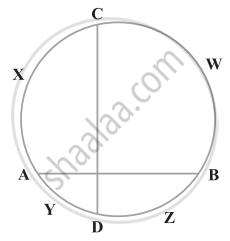Exercise 10.4 | Q 7 | Page 106

If ABC is an equilateral triangle inscribed in a circle and P be any point on the minor arc BC which does not coincide with B or C, prove that PA is angle bisector of ∠BPC.

Exercise 10.4 | Q 8 | Page 106

In figure, AB and CD are two chords of a circle intersecting each other at point E. Prove that ∠AEC = 1/2 (Angle subtended by arc CXA at centre + angle subtended by arc DYB at the centre).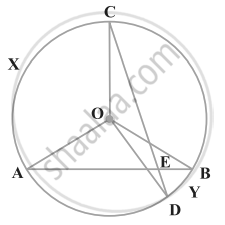Exercise 10.4 | Q 9 | Page 107

If bisectors of opposite angles of a cyclic quadrilateral ABCD intersect the circle, circumscribing it at the points P and Q, prove that PQ is a diameter of the circle.

Exercise 10.4 | Q 10 | Page 107

A circle has radius sqrt(2) cm. It is divided into two segments by a chord of length 2 cm. Prove that the angle subtended by the chord at a point in major segment is 45°.

Exercise 10.4 | Q 11 | Page 107

Two equal chords AB and CD of a circle when produced intersect at a point P. Prove that PB = PD.

Exercise 10.4 | Q 12 | Page 107

AB and AC are two chords of a circle of radius r such that AB = 2AC. If p and q are the distances of AB and AC from the centre, prove that 4q2 = p2 + 3r2.

Exercise 10.4 | Q 13 | Page 107

In figure,O is the centre of the circle, ∠BCO = 30°. Find x and y.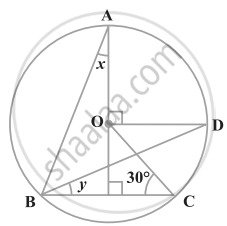Exercise 10.4 | Q 14 | Page 107

In figure, O is the centre of the circle, BD = OD and CD ⊥ AB. Find ∠CAB.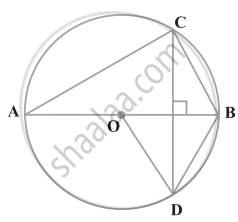## Chapter 10: Circles

Exercise 10.1Exercise 10.2Exercise 10.3Exercise 10.4## NCERT solutions for Mathematics Exemplar Class 9 chapter 10 - Circles

NCERT solutions for Mathematics Exemplar Class 9 chapter 10 (Circles) include all questions with solution and detail explanation. This will clear students doubts about any question and improve application skills while preparing for board exams. The detailed, step-by-step solutions will help you understand the concepts better and clear your confusions, if any. Shaalaa.com has the CBSE Mathematics Exemplar Class 9 solutions in a manner that help students grasp basic concepts better and faster.

Further, we at Shaalaa.com provide such solutions so that students can prepare for written exams. NCERT textbook solutions can be a core help for self-study and acts as a perfect self-help guidance for students.

Concepts covered in Mathematics Exemplar Class 9 chapter 10 Circles are Angle Subtended by a Chord at a Point, Perpendicular from the Centre to a Chord, Equal Chords and Their Distances from the Centre, Angle Subtended by an Arc of a Circle, Concept of Circle - Centre, Radius, Diameter, Arc, Sector, Chord, Segment, Semicircle, Circumference, Interior and Exterior, Concentric Circles, Cyclic Quadrilateral, Circles Passing Through One, Two, Three Points.

Using NCERT Class 9 solutions Circles exercise by students are an easy way to prepare for the exams, as they involve solutions arranged chapter-wise also page wise. The questions involved in NCERT Solutions are important questions that can be asked in the final exam. Maximum students of CBSE Class 9 prefer NCERT Textbook Solutions to score more in exam.

Get the free view of chapter 10 Circles Class 9 extra questions for Mathematics Exemplar Class 9 and can use Shaalaa.com to keep it handy for your exam preparation